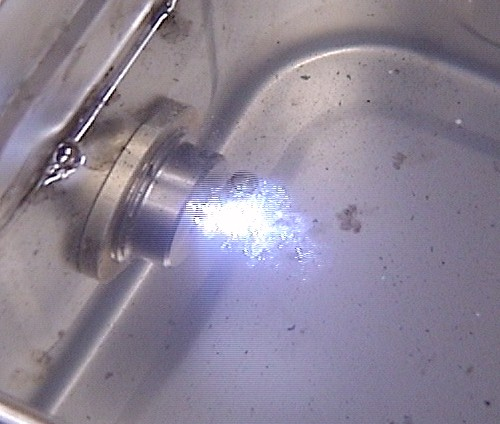#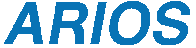ARIOS INC.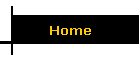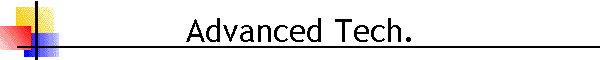## The multipoint Langmuir probe system

Introduction
Langmuir probe is the one of simple methods to measure plasma parameter, and can measure plasma and floating potential, electron and ion density, electron temperature and electron energy distribution function (EEDF). It is important for the plasma process to measure area distribution of plasma parameter. This multipoint Langmuir probe system was developed for this purpose.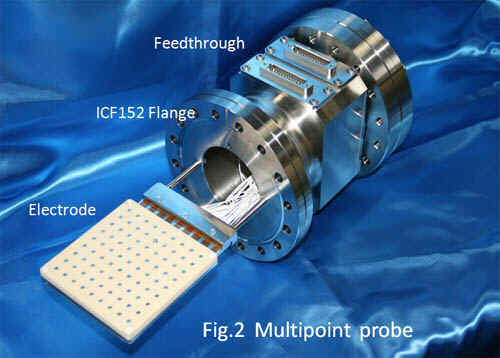Fig.1 is system diagram. multipoint probe photo shows Fig.2. This multipoint probe has 9x9=81 point probe on insulator board that the size is 100x100mm. These electrodes are connected to source measure unit (SMU) through vacuum feed through terminal and connection box. Connection box are selected each point probe and connected to SMU. Connection box is controlled by Personal Computer (PC).
SMU , which is Voltage Power Supply with ammeter, are used KeithleyTM 237(±1100V ±10mA) or YokogawaTM GS610(±110V ±500mA). GP-IB controller are used National InstrumentsTM (NI) GPIB-USB-HS.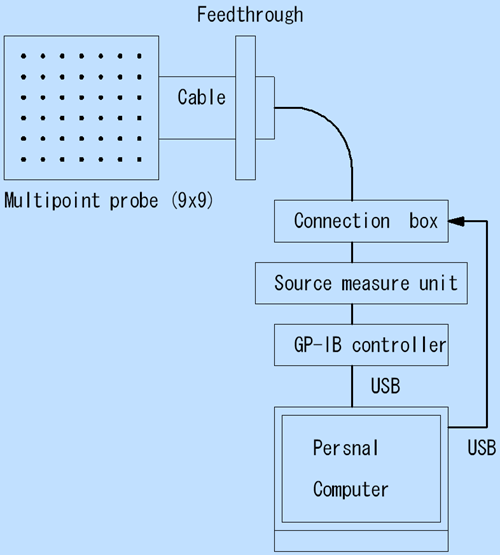Fig.1 Schematic of system diagram

A LabVIEW computer program was developed to run the data acquisition and the data analysis. Fig.3 shows operation screen.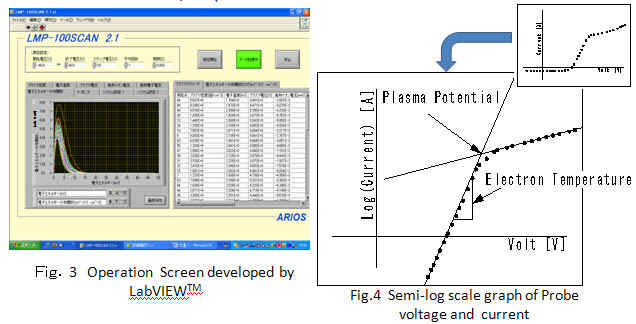There is two methods to determine the point of plasma potential from the voltage-current (V-I) data. One is differential calculus of the V-I curve. The maximum of the differential value is the point of plasma potential. This method outputs an accurate value, but is vulnerable to a noise. Other method is tangent line method. Exponential curve changes to straight line in semi-logarithmic V-I graph. The intersection of saturation region and exponential region is the point of plasma potential. As for this method, the answer includes error, but is provided surely. Tangent line method was adopted in this system. The tangent line was decided by using of Hough transform methods.
EEDF was obtained by double differential calculus of the V-I curve (Druyvestein Method ). This method is easy to be affected by noise. The interpretation of results needs carefulness.

These show the sample of results that was measured at the exit of remote plasma from microwave compact plasma source. Fig.5 shows the schematic of this plasma source. Inner diameter of aperture is 35mm. Fig.6 shows various plasma parameter at 200mm distance. In the plasma density, the highest center and a state of the diffusion can be observed. In all parameters, distribution can be recognized visually.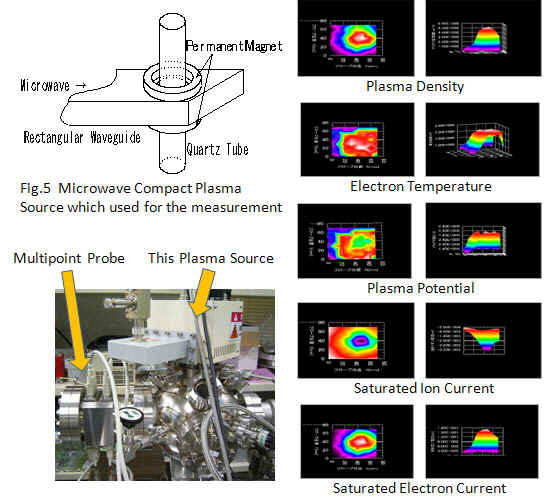Fig.7 shows EEDF. A difference of the electronic energy distribution can be observed.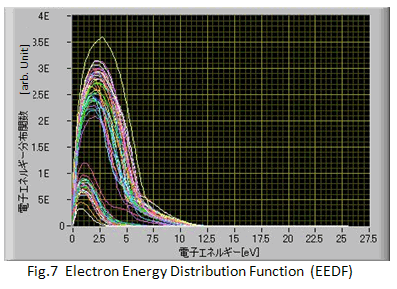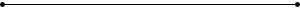## Homo-epitaxial CVD Diamond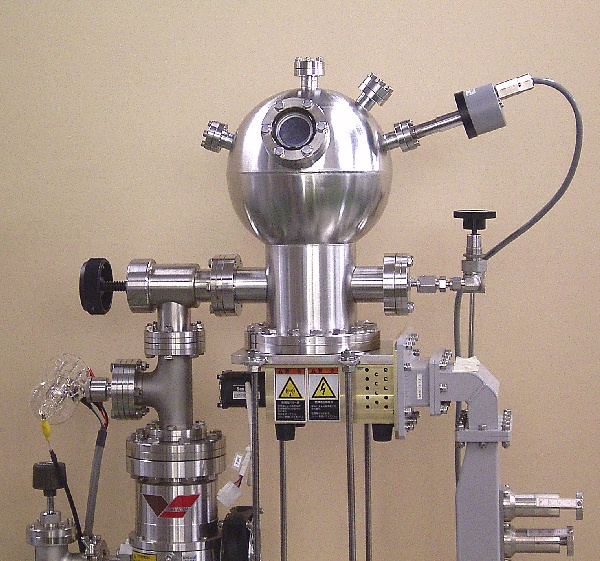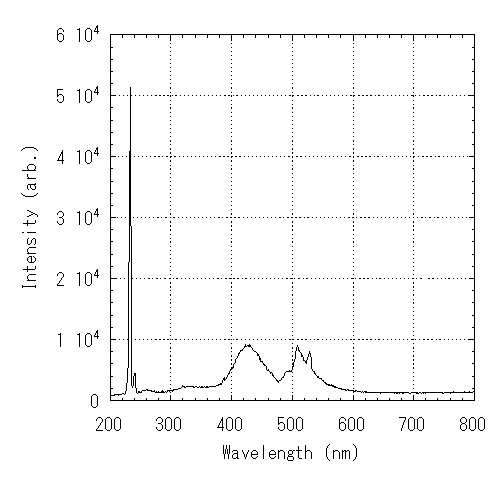Apparatus and cathode luminescence

Experimental conditions
7kPa, CH4 0.1% + H2, Microwave Power 400W, Sample Temperature 982 degree.## Plasma in water generated by microwave